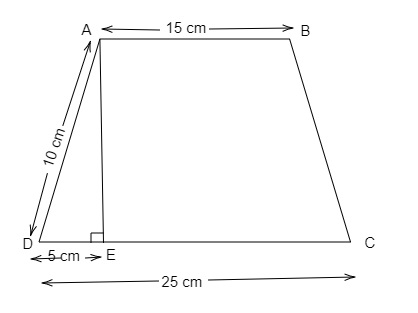# The parallel sides of a trapezium are 25 cm and 15 cm long. Its non-parallel sides are both equal, each being 10 cm long. Find the area of the trapezium.

Given:

The parallel sides of a trapezium are 25 cm and 15 cm long. Its non-parallel sides are both equal, each being 10 cm long.

To do:

We have to find the area of the trapezium.

Solution:Height of the trapeziumis AE.

$AD^2=AE^2+DE^2$

$10^2=AE^2+5^2$

$100=AE^2+25$

$AE^2=100-25=75$

$AE=\sqrt{75}=5\sqrt3$

We know that,

Area of a trapezium of height h and non-parallel sides a and b$=\frac{1}{2}\times h(a+b)$

$=\frac{1}{2}\times 5\sqrt3(25+15)$

$=\frac{1}{2}\times 5\sqrt3(40)$

$=100\sqrt3$

The area of the trapezium is $100\sqrt3$.

Updated on: 10-Oct-2022

30 Views